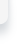Home Products Services Company Shop# Components! Calcualtor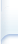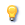Highlights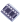Media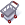Buy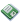Download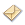Feedback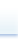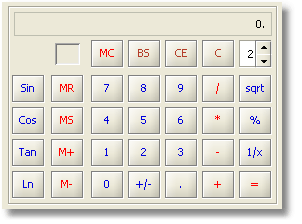The Calculator component is designed to perform any of the standard operations for which a user would normally use a handheld calculator. Calculator performs basic arithmetic, such as addition and subtraction, calculates reciprocal of the displayed number “1/x”, calculates the square root of the displayed number, and performs percentage calculations.   However, Calculator could easily be extended by the end-developer to perform any types of unary or binary calculations. In addition to calculation functionality, the Calculator component is able to store the displayed number in memory and allows several standard operations with the stored number: Recall stored number Clear Memory Add displayed number to the number already in memory Subtract displayed number from the number already in memory  When the user stores a number in the Calculator’s memory, an “ M” symbol appears in the box above the memory options. If the user stores another number, it replaces the one currently in memory. The Calculator component can present calculation results with different levels of precision – up to 11 decimal points. The number of fraction digits in the result field can be controlled by the Fraction Digits Spinner, however all internal calculations are always performed with an accuracy of 11 significant decimal digits!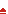Top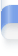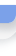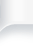Copyright © 2011 jProductivity, LLC